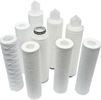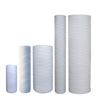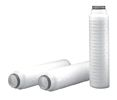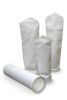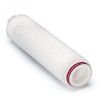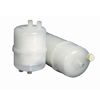1345
com_content.article
(0 votes, average 0 out of 5)
Các ??nh lu?t và công th?c tính tóan0 out of 50 based on 0 voters.

 Pascal’s Law Pressure exerted on a confined fluid is transmitted undiminished in all directions, and acts with equal force on all equal areas and at right angles to them.   Hydraulics Simply, a means of power transmission.   Work Force acting through distance. WORK = FORCE x DISTANCE Example: Work = lbs. x inches, or Force (lbs.) x Distance (ins.)   Power The rate of doing work.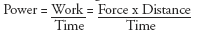Force   The Force (pounds) exerted by a piston can be determined by multiplying the piston area (sq. inches) by the pressure applied (psi). Force = Pressure x Area   Volume To determine volume (cubic inches) required to move a piston a given distance, multiply the piston cross sectional area (sq. inches) by the stroke required (inches). Volume = Area x L   Compression of Hydraulic Oil Hydraulic oil serves as an excellent lubricant, is practically non-compressible. It will compress approximately 0.4 of 1% at 1000 psi and 1.1% at 3000 psi at 1200. Weight of Hydraulic Oil The weight of hydraulic oil may vary with a change in viscosity; however, 55 to 58 lbs. per cubic foot covers the viscosity range from 150 SSU to 900 SSU at 1000?F.   Pressure in a Column of Oil The pressure at the bottom of a one-foot column of oil will be approximately 0.4 psi. To find the approximate pressure in psi at the bottom of any column of oil, multiply the height in feet by 0.4.   Atmospheric pressure Equivalent to 14.7 PSIA at sea level. ÄP means pressure difference.   Gage readings Gage readings do not include atmospheric pressure unless marked PSIA.   Pressure drop There must be a pressure drop (pressure difference) across an orifice or restriction to cause flow through it. Conversely, if there is no flow, there will be no pressure drop.   Pumps and fluids Fluid is pushed, not drawn, into a pump. If pumping from an open reservoir, atmospheric pressure pushes the fluid into the pump. Some pumps are used specifically to create pressure. Any resulting flow is incidental. A pump does not pump pressure; its purpose is to create flow. A pump used to transmit power is usually positive displacement type.   Pressure Pressure is caused by resistance to flow. A pressure gage indicates the pressure in a given unit, measured in PSI.Today 30
Tìm ki?m s?n ph?mHàng có s?n trong kho! Giá t?t khi mua s? l??ng l?n!